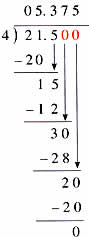Thursday , July 7 2022# NCERT 5th Class (CBSE) Mathematics: Decimals

## DIVISION IN DECIMAL NUMBERS

### A. Dividing by Whole Numbers

(a) Mrs. Sethi bought 4.35 kg of dry fruit for Diwali and distributed it equally among her three friends. How much did each friend get?

4.35 ÷ 3 = ? (divisor smaller than dividend)

Dividing by decimals is just like dividing whole numbers, except for placing the decimal point.

Step 1. Place the decimal point in the quotient directly above the decimal point.

3) 4.35 (← Decimal point directly above the decimal point in the dividend.

Step 2. Divide as if you were dividing whole numbers.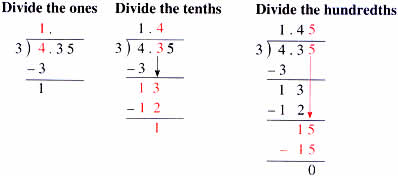Answer: Each friend gets 1.45 kg of dry fruit.

(b) 1.33÷7 ( divisor greater than dividend)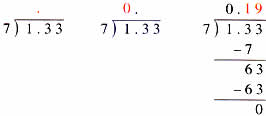Check:

0.19×7 = 1.33

Divisor×Quotient = Dividend

### B. Remainders While Dividing Decimals

(a) Shruti’s mother brought 3.5 m of cloth to make two dress. If the cloth was cut into two equal pieces, how long was each piece?

3.5÷2 = ?

2 ) 3.5 ( .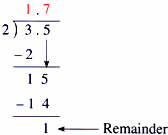When you divide decimals, you do not show remainders. You have to write extra zeros to complete the division.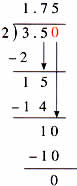Answer: Each piece was 1.75 m long.

(b) 1.26÷12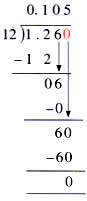(c) 21.5÷4 = ?

4 ) 21.5 ( .